# Acceleration convergence using a potential function

We cover the analysis of acceleration from this paper. The main motivation is to fill in some of the details that has been left out most likely for clarity.

### 1.1 Rewriting in terms of Mirror descent

We can write NAG:aswhere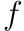is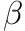-smooth,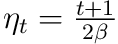and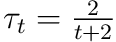(See e.g. this paper). This is the scheme we will be analysing.

### 1.2 Convergence

the general strategy is using potential function goes like this:

1. Define a potential function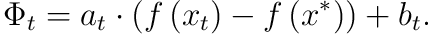1. Find bound for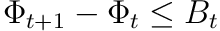.
2. Get convergence by telescoping the difference,##### Acceleration
• For acceleration we start with the potential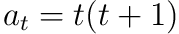and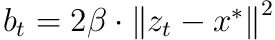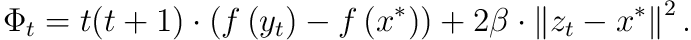This suggests that we will apply primal analysis to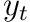and dual to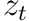.

• We want to bound the difference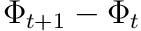which we can expand as• Using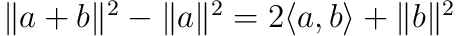(for euclidean norm) and the update forwe rewrite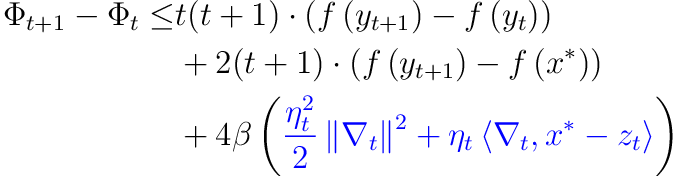Interpretation: we want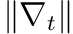to be small for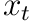to improve the most.

•-smooth and the update rule for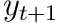gives usInterpretation: we wantto be large forto improve the most.

This lets us bound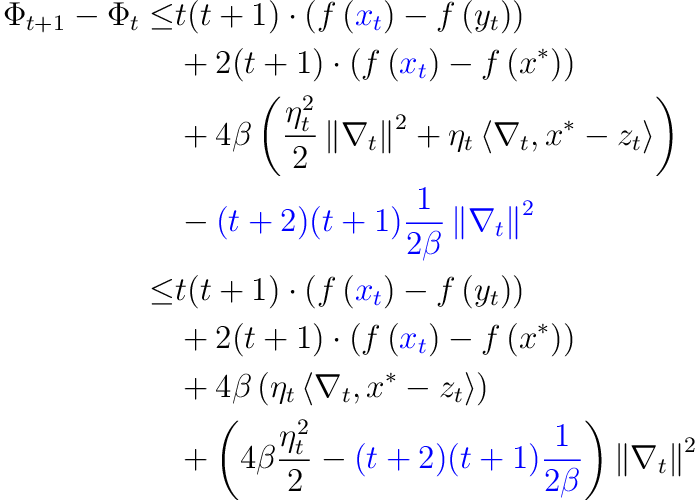By picking the slow step size to besuch that the last term disappears this reduces to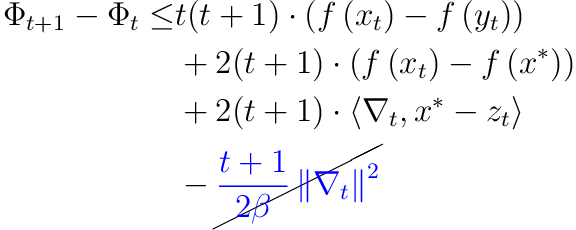• By convexity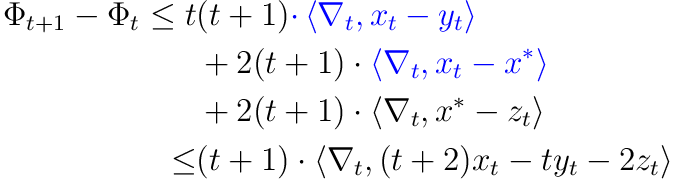• Using that the update is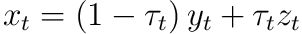we choose the step size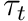such that the terms cancelsThe RHS of the inner product cancels,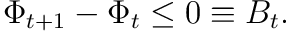• Now we have a bound for. We directly get from eq. 1So to be less than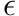we have to run for at least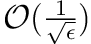iterations. This is a quadratic speed up over vanilla gradient descent on smooth functions which requires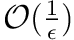steps.

The crucial observation is that we boundin terms of the norm andin terms of the gradient. This technique is further exploited in Mirr+Grad paper for general Mirror maps in the aggressive update,.